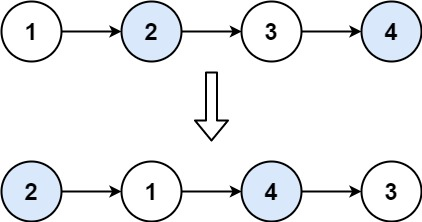24. Swap Nodes in Pairs

Example 1:```Input: head = [1,2,3,4]
Output: [2,1,4,3]
```

Example 2:

```Input: head = []
Output: []
```

Example 3:

```Input: head = 
Output: 
```

Constraints:

• The number of nodes in the list is in the range `[0, 100]`.
• `0 <= Node.val <= 100`

Follow up: Can you solve the problem without modifying the values in the list's nodes? (i.e., Only nodes themselves may be changed.)

24. Swap Nodes in Pairs
``````struct Solution;
use rustgym_util::*;

impl Solution {
if let Some(mut first) = head {
if let Some(mut second) = first.next.take() {
let rest = second.next.take();
first.next = Self::swap_pairs(rest);
second.next = Some(first);
Some(second)
} else {
Some(first)
}
} else {
None
}
}
}

#[test]
fn test() {
let head = list!(1, 2, 3, 4);
let res = list!(2, 1, 4, 3);